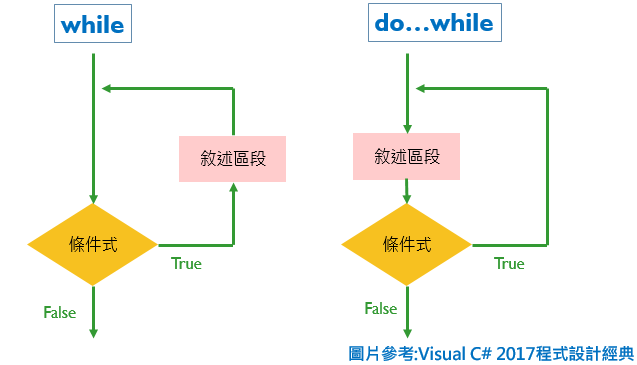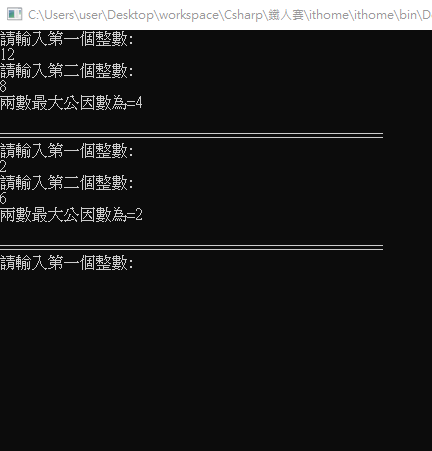#DAY 16
1
Modern Web

## Day16-C#- while與do..while(例:求因數、求最大公因數、怎麼讓console重複執行?)

1. while:前測試迴圈，通常用在當需要重複執行同樣動作，且`執行的次數為未知`時使用。也就是把條件判斷放在前面，先判斷條件符不符合，符合則執行迴圈。
2. do...while:後測試迴圈，與while相同，但是第一次進入迴圈時不用檢查是否滿足條件，先執行一次迴圈內容後，第二次再判斷是否符合條件，符合條件再次進入迴圈。也就是這個寫法`迴圈不管如何至少會執行一次`# while

while(條件)
{

}

``````Console.WriteLine("請輸入整數N:");
int factor = 1;
while (factor <= N)//欲判斷的因數是否<=N
{
if (N % factor == 0)//如果可以整除N代表factor是N的因數
{
}
factor++;//每次執行完+1
}
``````

``````int a, b, c, g;
Console.WriteLine("請輸入第一個整數:");
Console.WriteLine("請輸入第二個整數:");
if (b > a)//把兩數比較大的數換到被除數
{
(a, b) = (b, a);//把兩個數互換
}
while (a % b != 0)//兩個數相除不為0,代表還能繼續除
{
g = a % b;//g為餘數
a = b;//把除數變成被除數
b = g;//把餘數變成除數
}
Console.WriteLine(\$"兩數最大公因數為={b}");
``````

``````while (true)//這樣寫代表無窮迴圈，這裡表示只要條件式是true就一直執行while迴圈
{
int a, b, c, g;
Console.WriteLine("請輸入第一個整數:");
Console.WriteLine("請輸入第二個整數:");
if (b > a)//把兩數比較大的數換到被除數
{
(a, b) = (b, a);//把兩個數互換
}
while (a % b != 0)//兩個數相除不為0,代表還能繼續除
{
g = a % b;//g為餘數
a = b;//把除數變成被除數
b = g;//把餘數變成除數
}
Console.WriteLine(\$"兩數最大公因數為={b}");
Console.WriteLine("");
Console.WriteLine("================================================");
}
``````do
{

}while(條件);

# 參考資料

Visual C＃ 2017程式設計經典